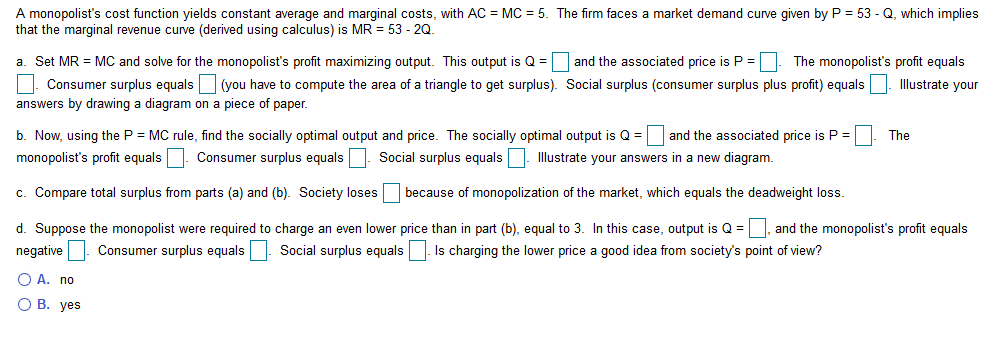# A monopolist's cost function yields constant average and marginal costs, with AC MC 5. The firm faces a market demand curve given by P = 53 - Q, which impliesthat the marginal revenue curve (derived using calculus) is MR = 53 - 2Q.a. Set MRMC and solve for the monopolist's profit maximizing output. This output is Qand the associated price is P =| The monopolist's profit equalsConsumer surplus equals(you have to compute the area of a triangle to get surplus). Social surplus (consumer surplus plus profit) equalsllustrate youranswers by drawing a diagram on a piece of paper.and the associated price is P =b. Now, using the P MC rule, find the socially optimal output and price. The socially optimal output is Q=TheConsumer surplus equalsIllustrate your answers in a new diagrammonopolist's profit equalsSocial surplus equalsc. Compare total surplus from parts (a) and (b). Society losesbecause of monopolization of the market, which equals the deadweight lossd. Suppose the monopolist were required to charge an even lower price than in part (b), equal to 3. In this case, output is Qand the monopolist's profit equalsSocial surplus equalsIs charging the lower price a good idea from society's point of view?Consumer surplus equalsnegativeO A. noО В. уes

Question
52 views

Part A, B, Chelp_outlineImage TranscriptioncloseA monopolist's cost function yields constant average and marginal costs, with AC MC 5. The firm faces a market demand curve given by P = 53 - Q, which implies that the marginal revenue curve (derived using calculus) is MR = 53 - 2Q. a. Set MR MC and solve for the monopolist's profit maximizing output. This output is Q and the associated price is P =| The monopolist's profit equals Consumer surplus equals(you have to compute the area of a triangle to get surplus). Social surplus (consumer surplus plus profit) equals llustrate your answers by drawing a diagram on a piece of paper. and the associated price is P = b. Now, using the P MC rule, find the socially optimal output and price. The socially optimal output is Q= The Consumer surplus equals Illustrate your answers in a new diagram monopolist's profit equals Social surplus equals c. Compare total surplus from parts (a) and (b). Society losesbecause of monopolization of the market, which equals the deadweight loss d. Suppose the monopolist were required to charge an even lower price than in part (b), equal to 3. In this case, output is Q and the monopolist's profit equals Social surplus equalsIs charging the lower price a good idea from society's point of view? Consumer surplus equals negative O A. no О В. уes fullscreen
check_circle

Step 1

* Hello. Since your question has multiple sub-parts, we will solve first three sub-parts for you. If you want remaining sub-parts to be solved, then please resubmit the whole question and specify those sub-parts you want us to solve.

Given Information:

AC = MC = 5

Demand Function: P = 53 - Q

MR = 53 - 2Q

Step 2
1.

MR = MC

53 - 2Q = 5

Q =24

P = 53 - Q

= 53 - 24

= 29

Profit = Total Revenue - Total Cost

= P * Q - AC * Q

= 29 * 24 - 5 * 24

= 576

Consumer Surplus (CS) is the area of triangle ABC.

(CS) = 1/2 * 24 * (53 - 29)

= 288

Social Surplus(SS) = Profit + CS

= 576 + 288

= 864

The diagram showing the results is shown below:

Step 3
1.

P = MC

53 - Q = 5

Q = 48

P = 53 - Q

= 5

Profit = Total Revenue - Total Cost

= P * Q - AC * Q

= 5 * 48 - 5 * 48

= 0

Consumer Surplus (CS) is the area of triangle DEF.

(CS) = 1/2 * 48 * (53 - 5)

= 1152

...

### Want to see the full answer?

See Solution

#### Want to see this answer and more?

Solutions are written by subject experts who are available 24/7. Questions are typically answered within 1 hour.*

See Solution
*Response times may vary by subject and question.
Tagged in